BROWSE BY

#### Author

Results 1 - 10 of 19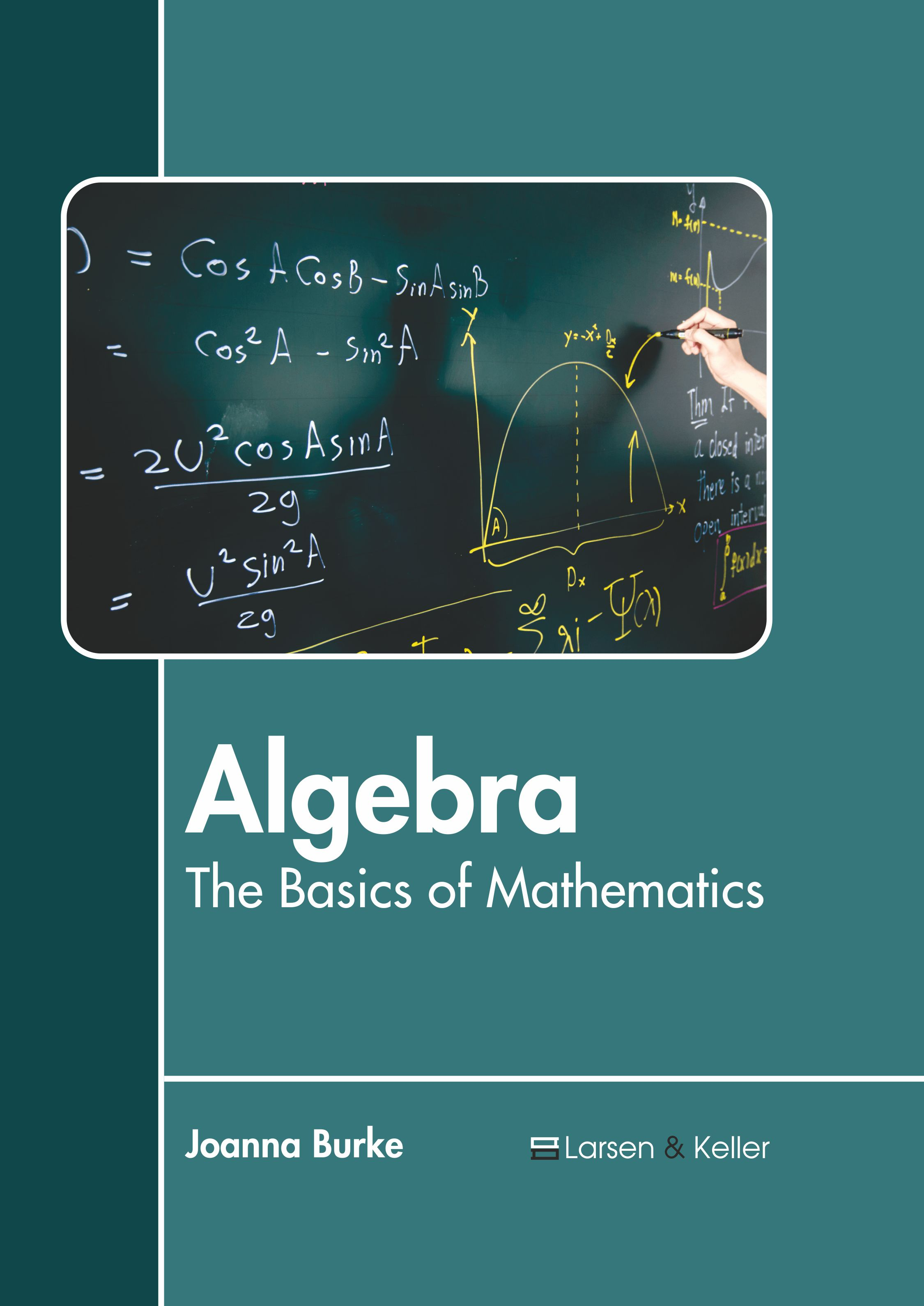Algebra: The Basics of Mathematics Author : Joanna Burke Subject : Mathematics and Statistics ISBN :9781641724920 Algebra is one of the major areas of study in mathematics, along with geometry, number theory and analysis. Algebra studies mathematical symbols as well as the regulations which govern their manipulation. Read More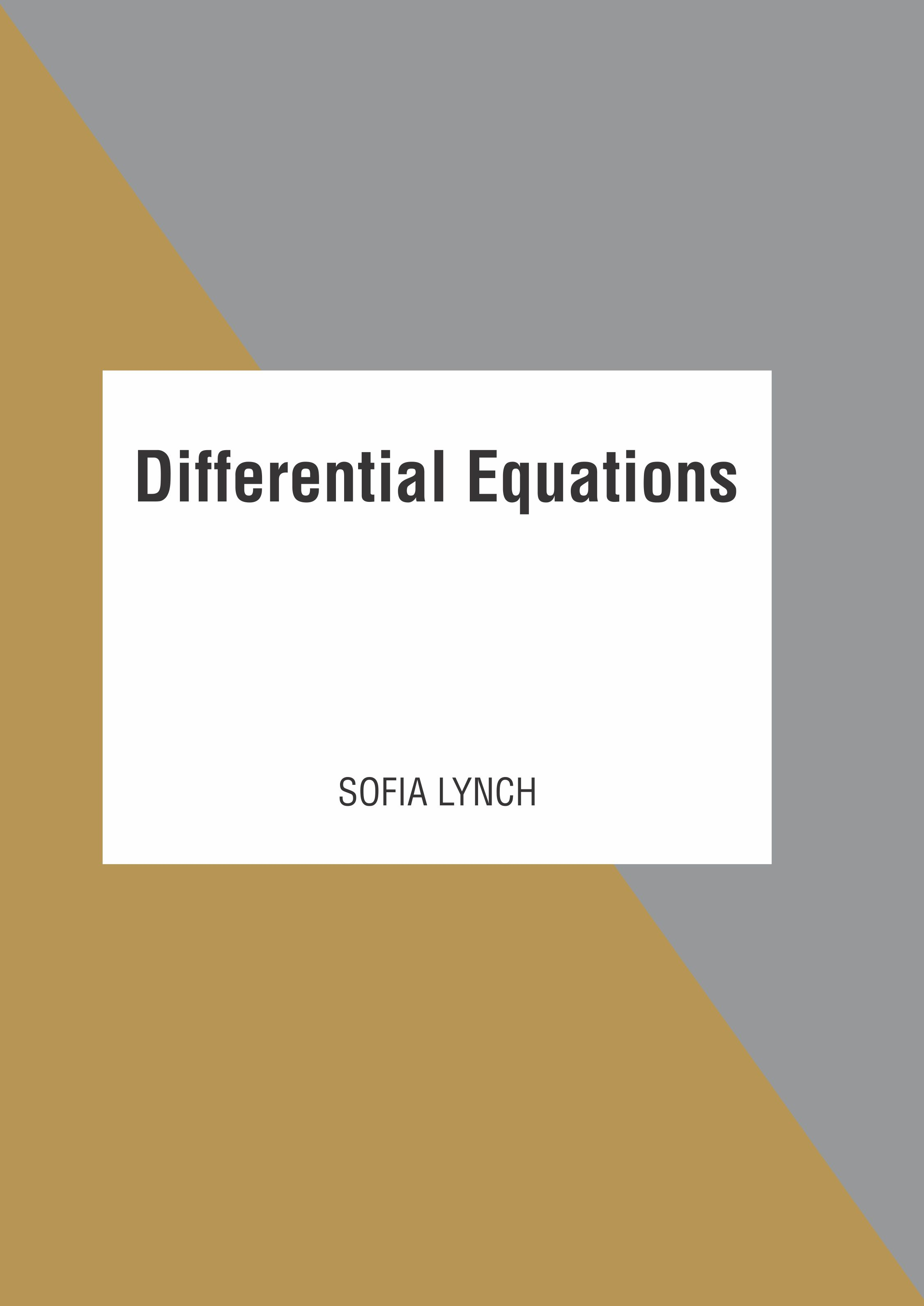Differential Equations Author : Sofia Lynch Subject : Mathematics and Statistics ISBN :9781641724937 A mathematical equation which relates some function with its derivatives is known as a differential equation. While applying a differential equation, the physical quantities are represented by functions Read More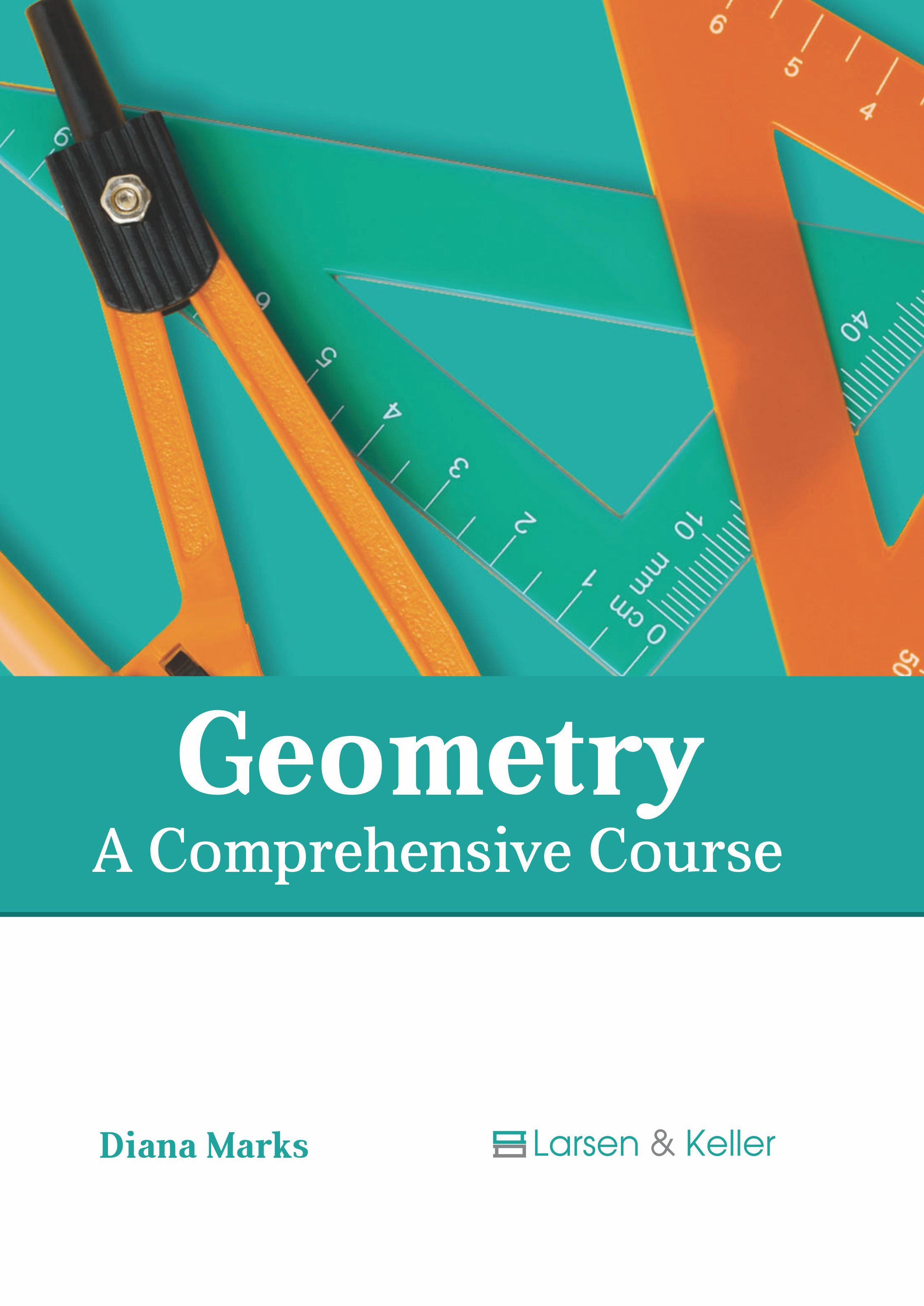Geometry: A Comprehensive Course Author : Diana Marks Subject : Mathematics and Statistics ISBN :9781641724029 Geometry is a mathematical branch that deals with the questions of size, shape, relative position of figures and the properties of space. Some of the fundamental concepts of geometry include concepts of Read More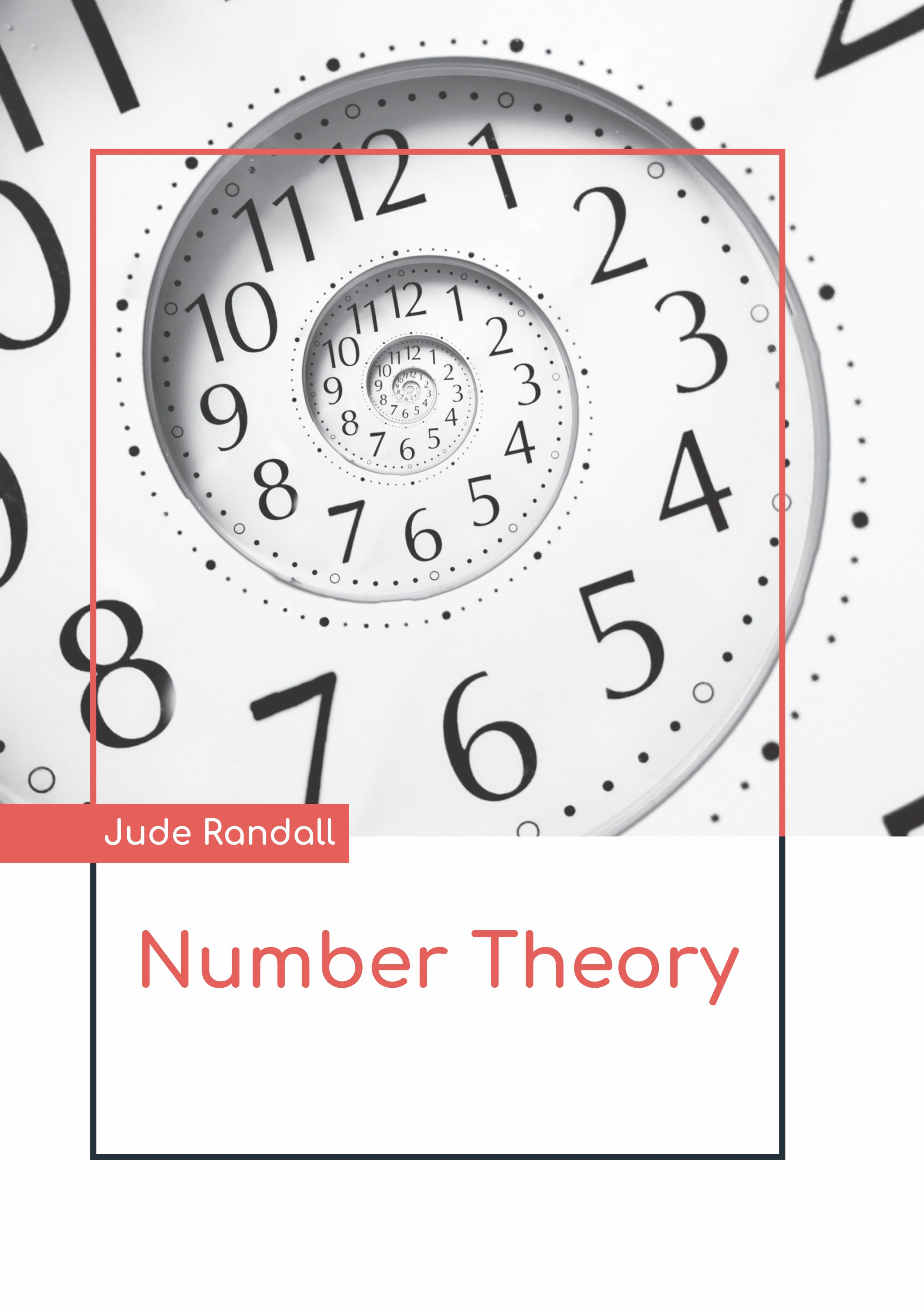Number Theory Author : Jude Randall Subject : Mathematics and Statistics ISBN :9781641724159 The branch of pure mathematics that primarily deals with the study of the integers and integer-valued functions is referred to as number theory. It includes the study of prime numbers as well as the properties Read More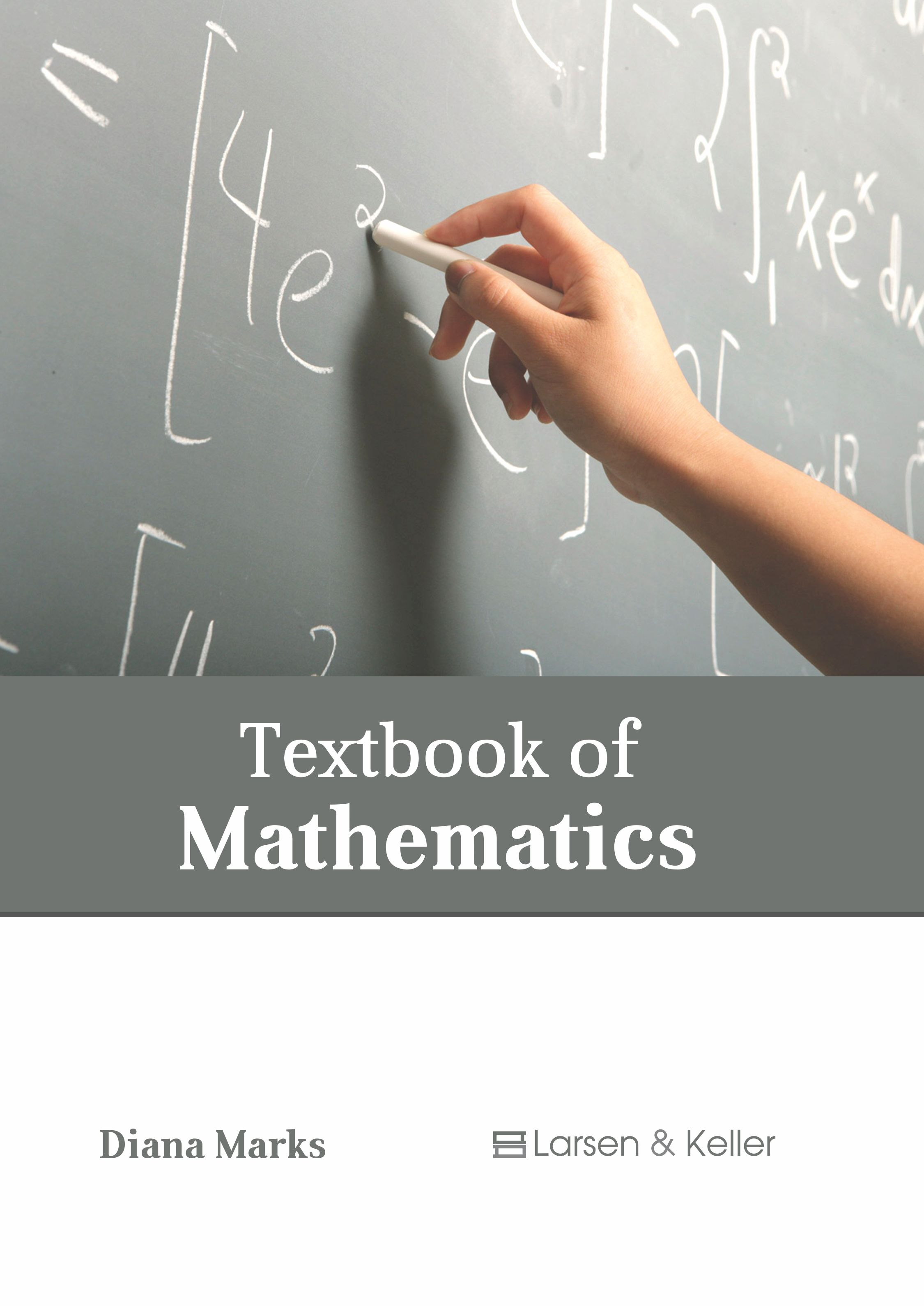Textbook of Mathematics Author : Diana Marks Subject : Mathematics and Statistics ISBN :9781641724036 The discipline of mathematics is concerned with the study of topics such as quantity, space, change and structure. It uses patterns for the formulation of new conjectures. Pure mathematics and applied Read More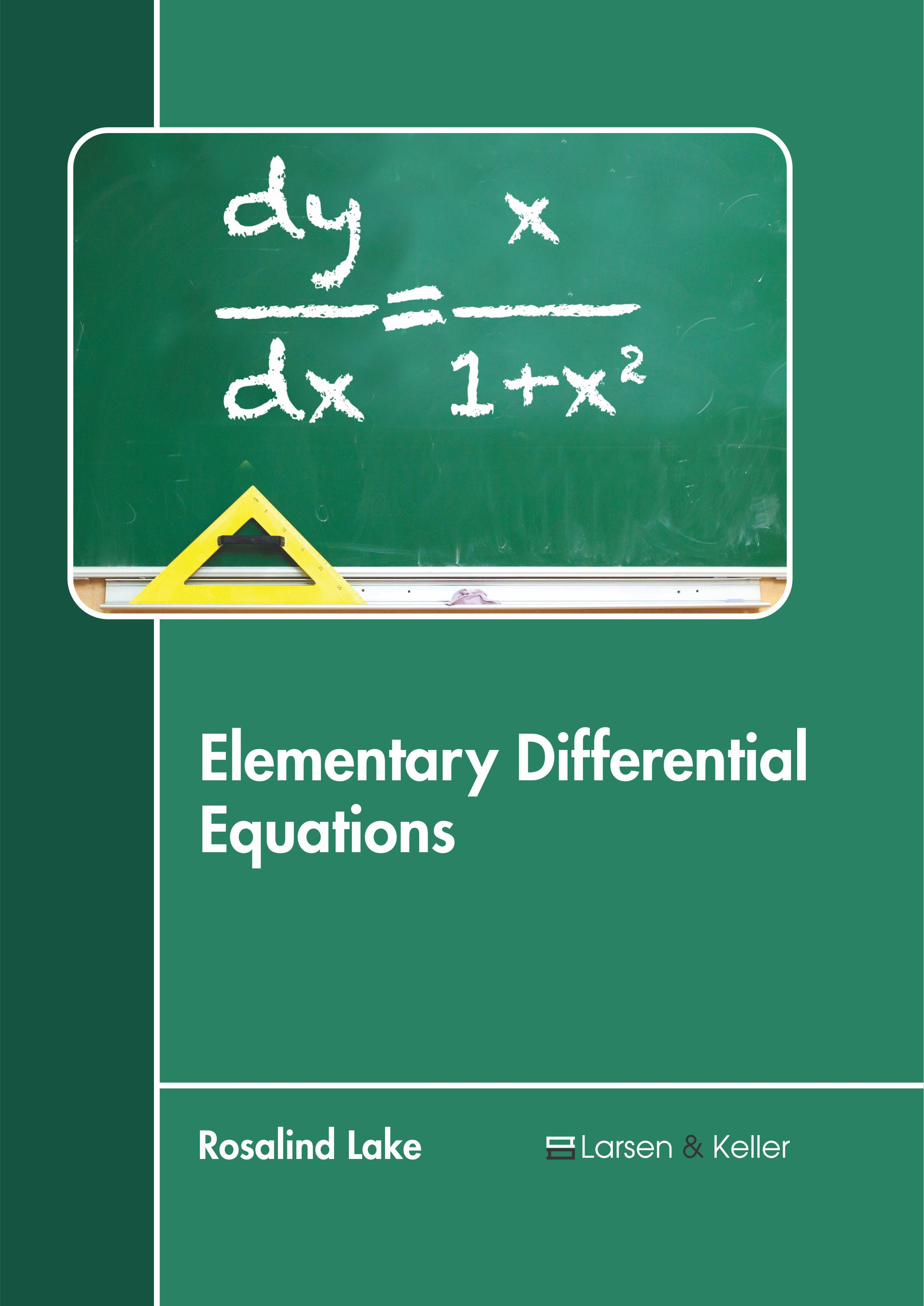Elementary Differential Equations Author : Rosalind Lake Subject : Mathematics and Statistics ISBN :9781641721219 A differential equation is a mathematical equation containing functions and their derivatives. A function represents a physical quantity, its derivative expresses the rate of change and the equation describes Read More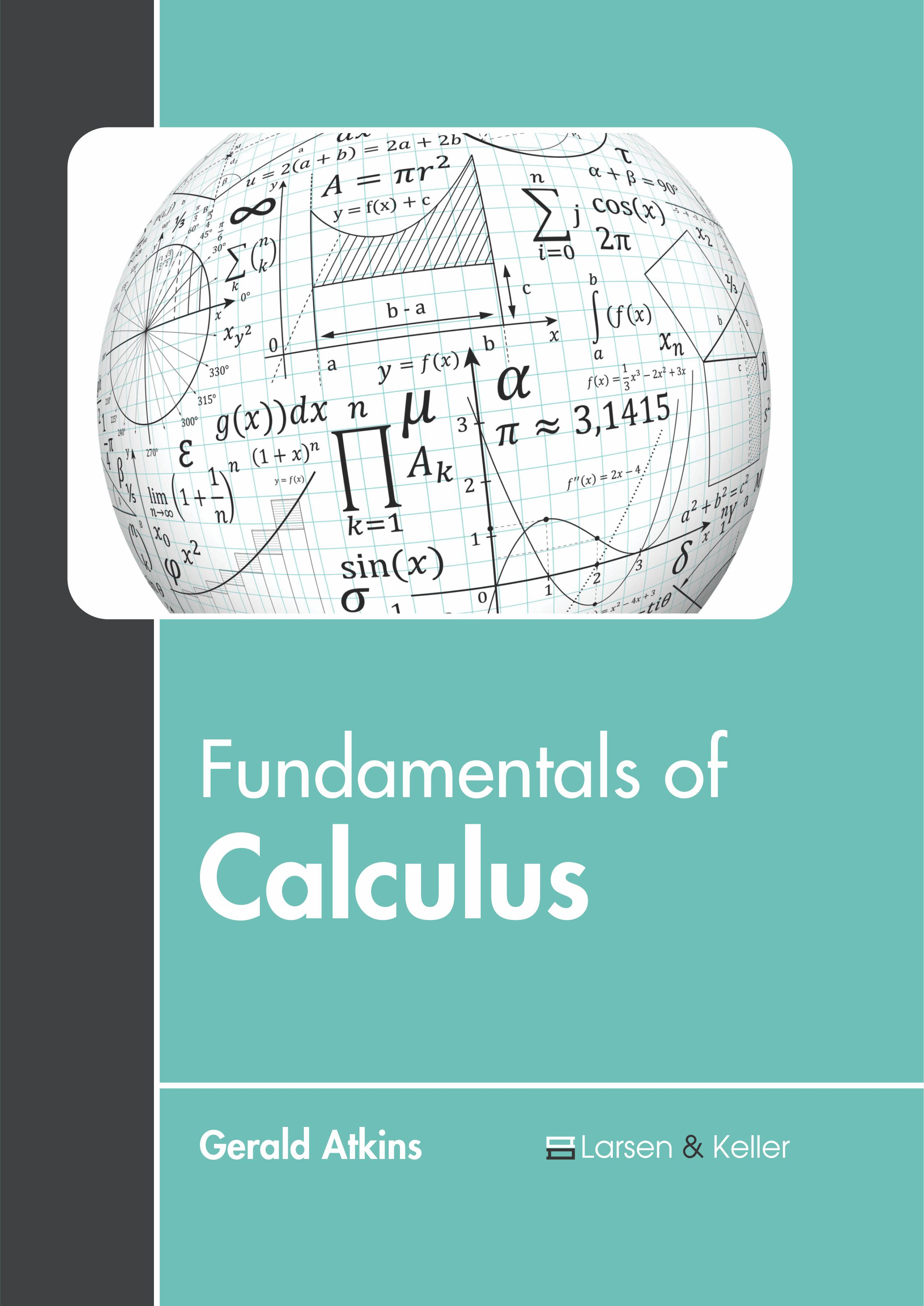Fundamentals of Calculus Author : Gerald Atkins Subject : Mathematics and Statistics ISBN :9781641721400 Calculus is a branch of mathematics that studies continuous change. It can be divided into the two branches of differential and integral calculus. The principles of limits and infinitesimals, the fundamental Read More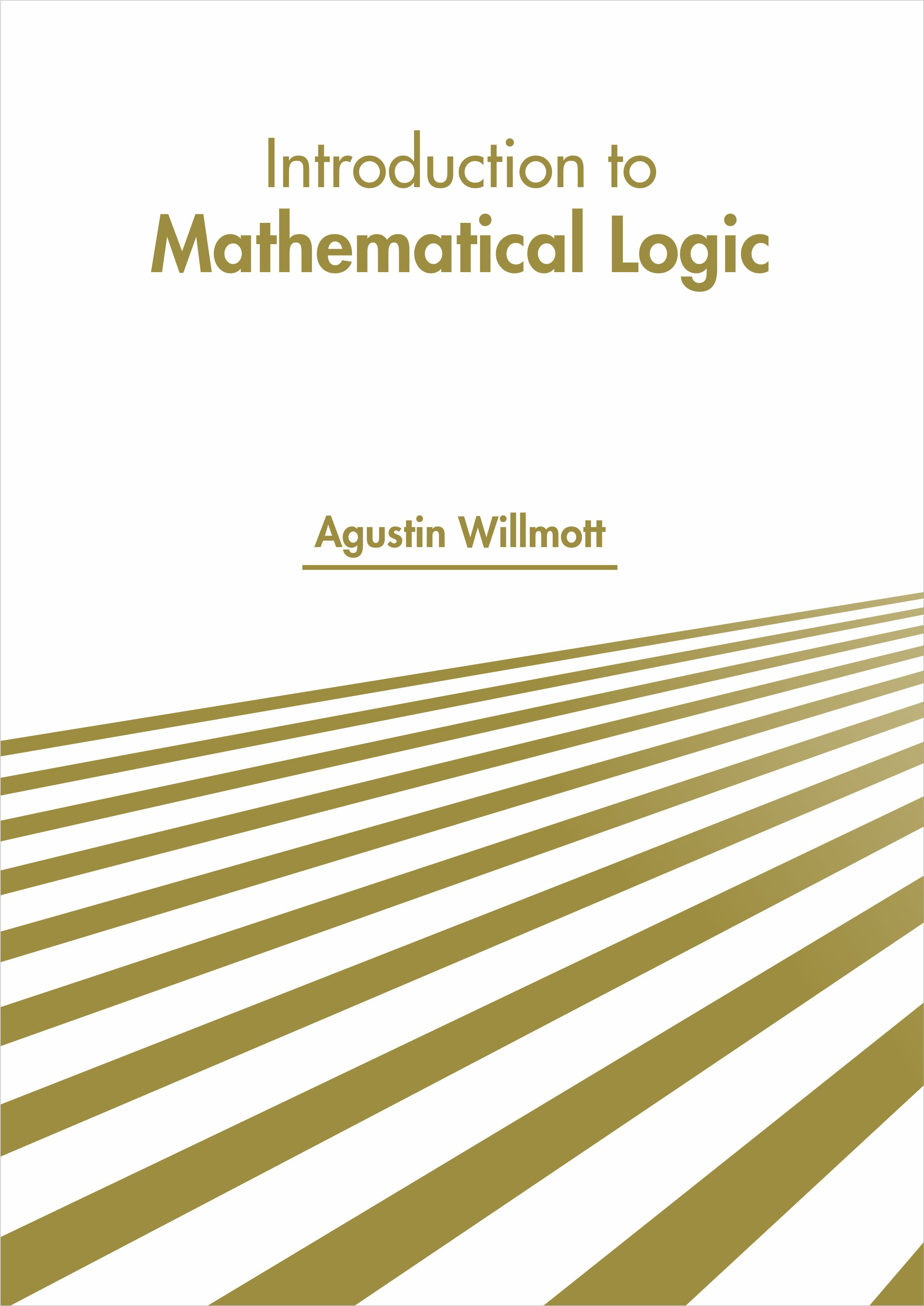Introduction to Mathematical logic Author : Agustin Willmott Subject : Mathematics and Statistics ISBN :9781641721004 Mathematical logic is a subfield of mathematics that is concerned with the application of formal logic to mathematics. It is closely associated with the foundations of mathematics, metamathematics and Read More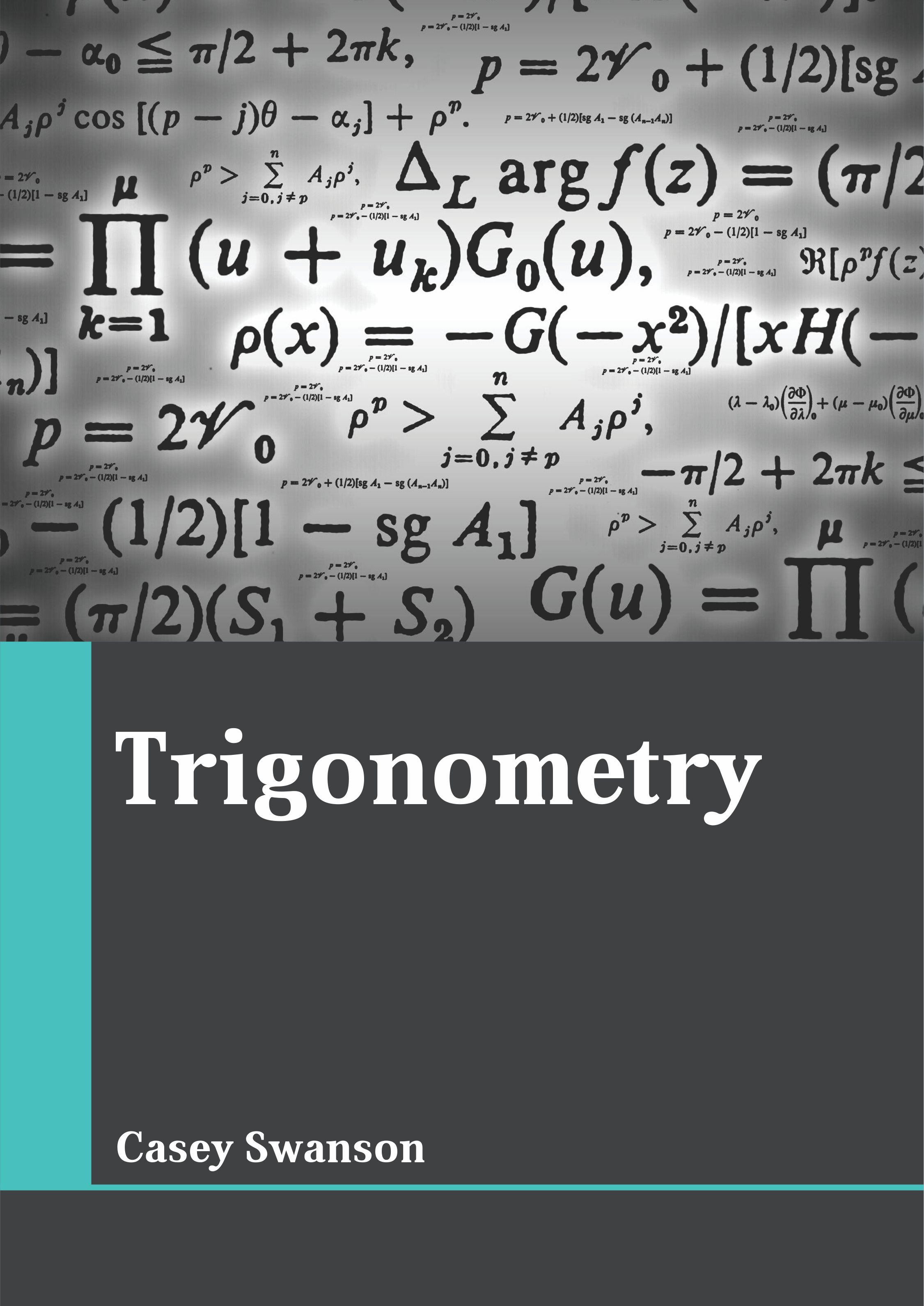Trigonometry Author : Casey Swanson Subject : Mathematics and Statistics ISBN :9781641721356 Trigonometry is an important field of mathematics. It deals with the study of relationship between angles and lengths of triangles. Such studies have led to the development of trigonometric functions. Read More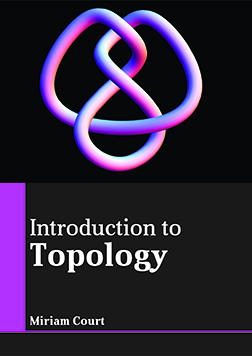Introduction to Topology Author : Miriam Court Subject : Mathematics and Statistics ISBN :9781635497045 Topology is a sub-field of mathematics. It deals with the study of preserved properties of space under repeated crumpling, stretching and bending. This area includes two major properties namely compactness, Read More
Results 1 - 10 of 19